# Divide 11

Divide the product of 4 and 5/8 by 1 1/2. Write your answer as a mixed number.

x =  1.6667

### Step-by-step explanation: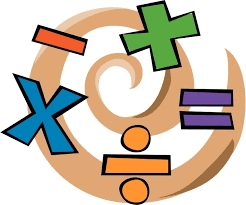Did you find an error or inaccuracy? Feel free to write us. Thank you!Tips to related online calculators
Need help to calculate sum, simplify or multiply fractions? Try our fraction calculator.

## Related math problems and questions:

• Divide 13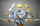Divide. Simplify your answer and write as an improper fraction or whole number. 14÷8/3
• DozenWhat is the product of 26 and 5? Write the answer in an Arabic numeral. Add up the digits. How many of this is in a dozen? Divide #114 by this
• Numbers divisionWith what number should be divided mixed number 2 3/4 to get 11/12?
• Evaluate 17Evaluate 2x+6y when x=- 4/5 and y=1/3. Write your answer as a fraction or mixed number in simplest form.
• Equivalent fractions 2Write the equivalent multiplication expression. 2 1/6÷3/4
• A turtle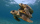A turtle is 20 5/6 inches below the surface of a pond. It dives to a depth of 32 1/4 inches. What is the change in the turtle’s position? Write your answer as a mixed number.
• Each year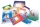Each year, Mr. Baumann purchases enough paper for his 16 students. If each student will use 3/4 pack of paper, how many packs of paper will he need to purchase? Answer as a mixed number or as a whole number.
• Fractions and mixed numerals(a) Convert the following mixed numbers to improper fractions. i. 3 5/8 ii. 7 7/6 (b) Convert the following improper fraction to mixed number. i. 13/4 ii. 78/5 (c) Simplify these fractions to their lowest terms. i. 36/42 ii. 27/45 2. evaluate following ex
• Two numbers and its productThe product of two numbers are 2/3. If on of them is 1/10, what is the other?
• A 14.5-gallonA 14.5-gallon gasoline tank is 3/4 full. How many gallons will it take to fill the tank? Write your answer as a mixed number.
• Division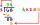Divide by the number 0.2 is the same as multiply by what number?
• Write 3Write a real world problem involving the multiplication of a fraction and a whole number with a product that is between 8 and 10 then solve the problem
• Quotient and productIf the quotient of [8/5 divided by 8/10] is added to the product of [8/14 x 7/12 x 3/8], what is the sum?
• Evaluate 11Multiply the quotient of 6 and 2 by 3, then add 1. Add 1 and 6, then divide by 2 and multiply by 3. Divide 6 by the product of 2 and 3, then add 1.
• DivideDivide the number 72 in the ratio 7: 2 and calculate the ratio of the numbers found in this order and write down as decimal.
• JonahJonah has an 8-pound bag of potting soil. He divides it evenly among 5 flowerpots. How much soil is in each pot? Show your answer as a fraction or mixed number. Solve this problem any way you choose.
• Unknown number4/5 of a number is 276. what is 2/3 of the same number?